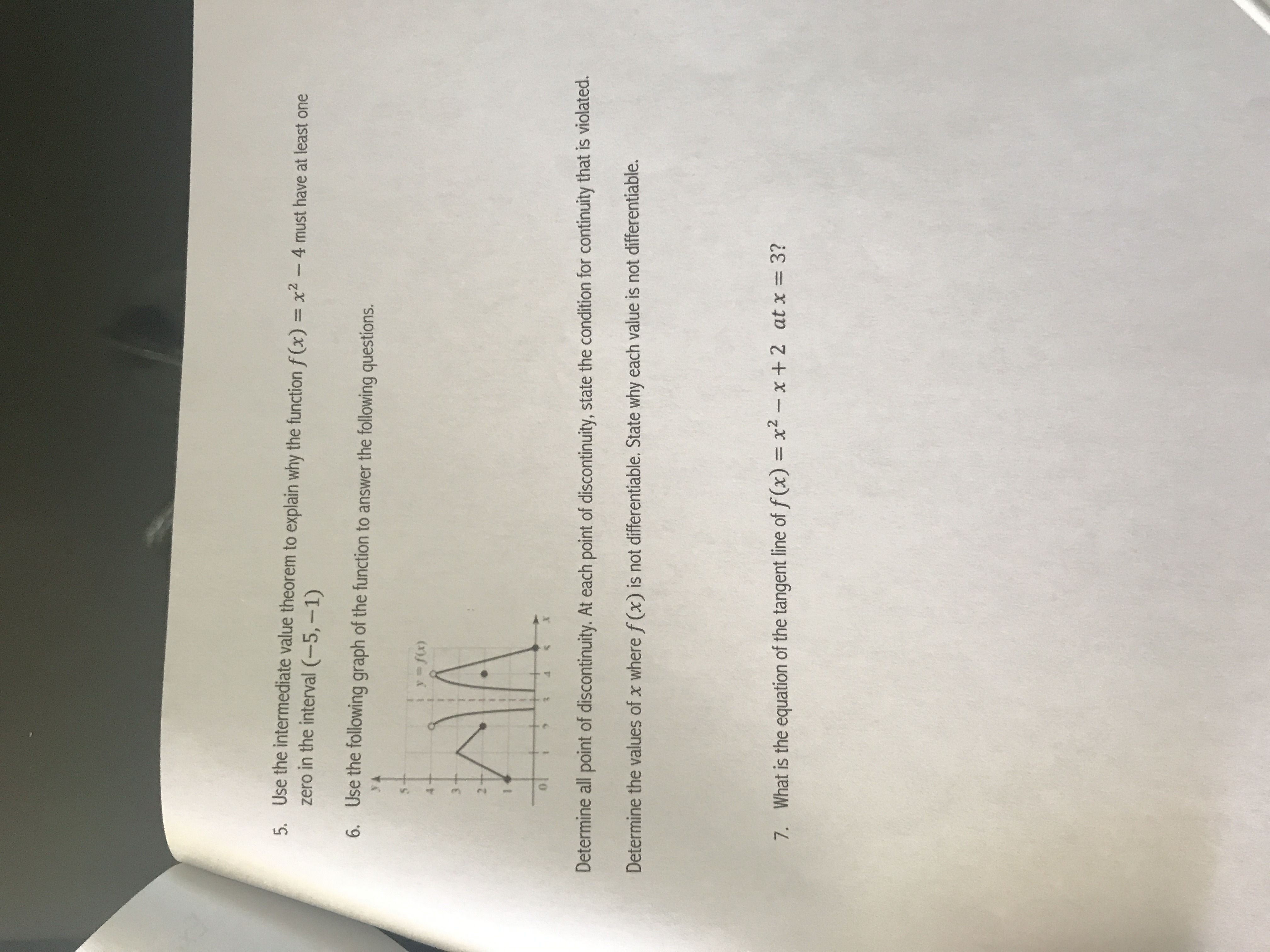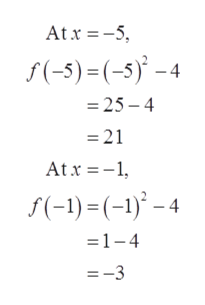Use the intermediate value theorem to explain why the function f(x) = x2 - 4 must have at least onezero in the interval (-5,-1)5.Use the following graph of the function to answer the following questions.6.HAyfix)Determine all point of discontinuity. At each point of discontinuity, state the condition for continuity that is violated.Determine the values of x where f (x) is not differentiable. State why each value is not differentiable.What is the equation of the tangent line of f(x) x2-x + 2 at x = 3?7.

Questionhelp_outlineImage TranscriptioncloseUse the intermediate value theorem to explain why the function f(x) = x2 - 4 must have at least one zero in the interval (-5,-1) 5. Use the following graph of the function to answer the following questions. 6. HA yfix) Determine all point of discontinuity. At each point of discontinuity, state the condition for continuity that is violated. Determine the values of x where f (x) is not differentiable. State why each value is not differentiable. What is the equation of the tangent line of f(x) x2-x + 2 at x = 3? 7. fullscreen
Step 1

We’ll answer the first question that is question 5 since the exact one wasn’t

specified. Please submit a new question specifying the one you’d like answered.

To apply intermediate value theorem to the function f(x) = x2 – 4 and determine why the function must have at least one zero in the interval (-5, -1).

Step 2

The intermediate value theorem states that for a function continuous f(x) on the interval [a, b] and a real number u lying between the interval  f(a) < u < f(b), there exists a constant c in the interval (a, b) such that f(c) = u.

Step 3

Consider the function f(x) = x‑2 – 4 is a polynomial function which is continuous on the interval [-5, -1].

Now...help_outlineImage TranscriptioncloseAt x 5 f(-5)=(-5)-4 =25- 4 =21 Atx1 f(-1)(-1)-4 1-4 =-3 fullscreen

Want to see the full answer?

See Solution

Want to see this answer and more?

Our solutions are written by experts, many with advanced degrees, and available 24/7

See Solution
Tagged in

Functions